# Introduction to Finite-Difference Time-Domain Method

This course is an introduction to the Finite-Difference Time-Domain (FDTD) Method for Electromagnetics. We will walk you through the basics of setting up and running electromagnetic simulations using the finite-difference time-domain (FDTD) method. Through this course, you will gain a knowledge of the fundamental concepts behind electromagnetic simulation as well as many advanced topics worth considering when you set up your simulations.

The course will use Flexcompute's Tidy3D solver for its examples and the scripts used in the slides are publicly accessible from this website. A basic knowledge of electromagnetics and programming is recommended to get the most use out of this course.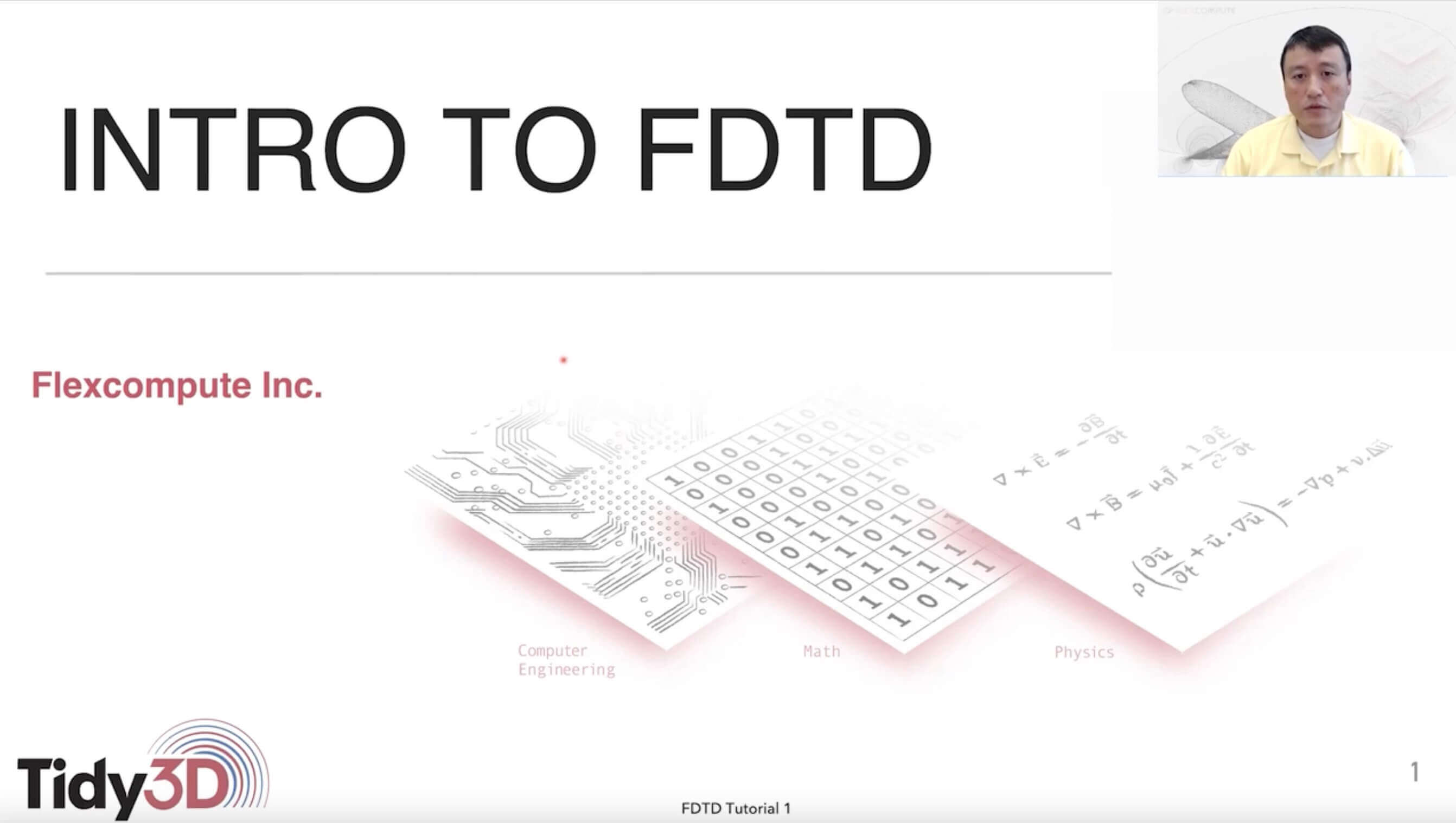## Lecture 1: Introduction to FDTD Simulation

In this lecture, we will describe the basic concepts of EM simulation using FDTD.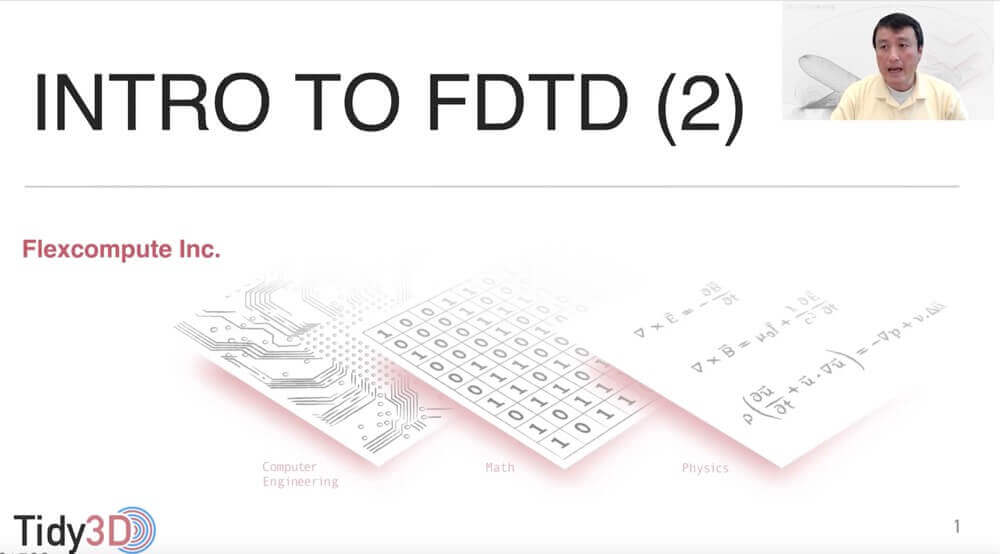## Lecture 2: Using FDTD to Compute a Transmission Spectrum

In this lecture, we show how to use FDTD to solve a basic EM problem involving the transmission of light though a slab of material.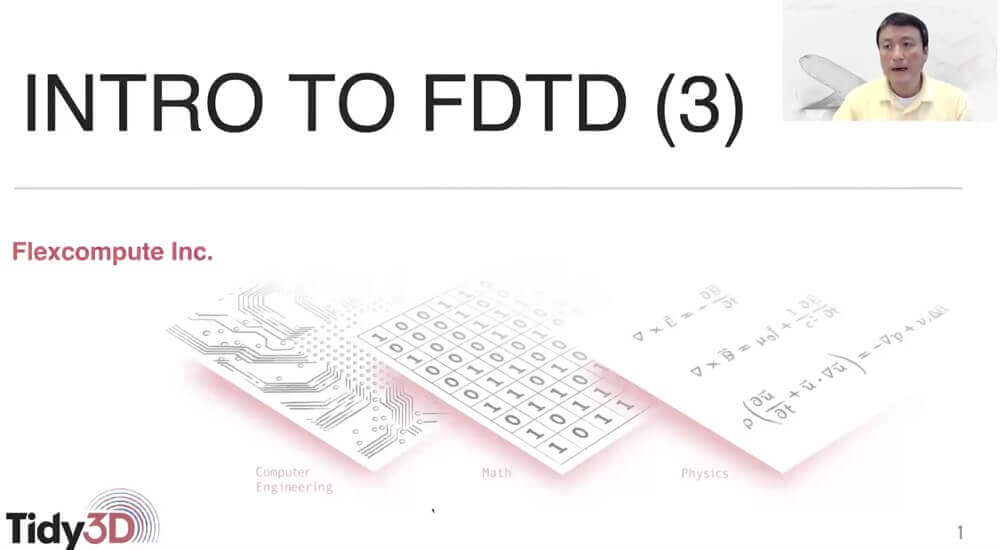## Lecture 3: Applying FDTD to Photonic Crystal Slab Simulation

In this lecture, we show how to apply FDTD to solve a slightly more complicated EM problem involving a photonic crystal slab that supports guided resonance.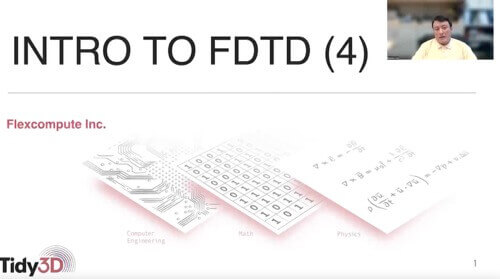## Lecture 4: Prelude to Integrated Photonics Simulation: Mode Injection

In this lecture, we show how to perfectly inject a specific mode into a straight waveguide. This is helpful in decreasing computational cost, and having a clean simulation without unwanted electromagnetic interference in the computational domain.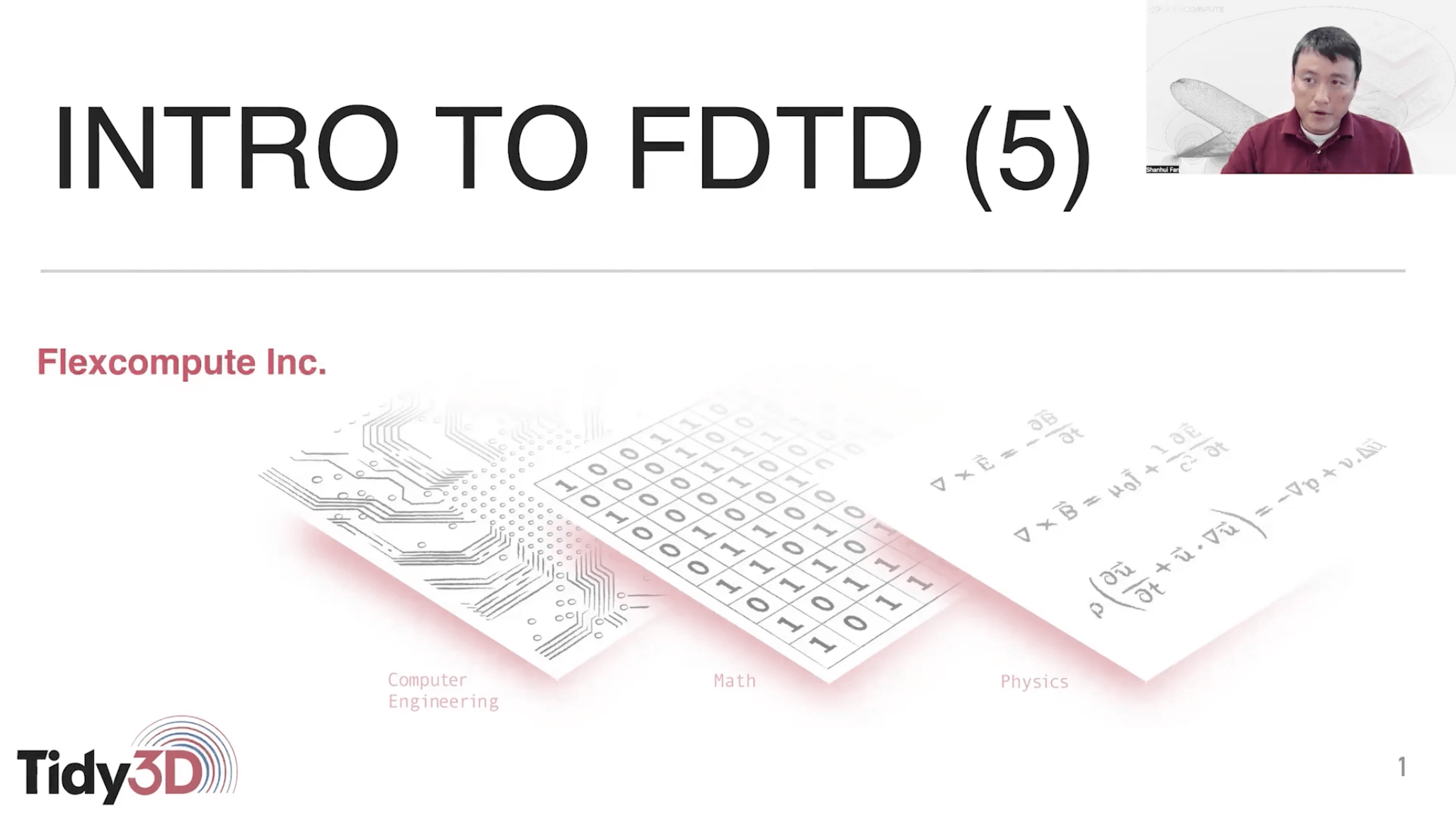## Lecture 5: Modeling dispersive material in FDTD

Material dispersion is a very common phenomenon in which the material responds differently to light of different color. In this lecture, we show how to include material dispersion in FDTD simulations.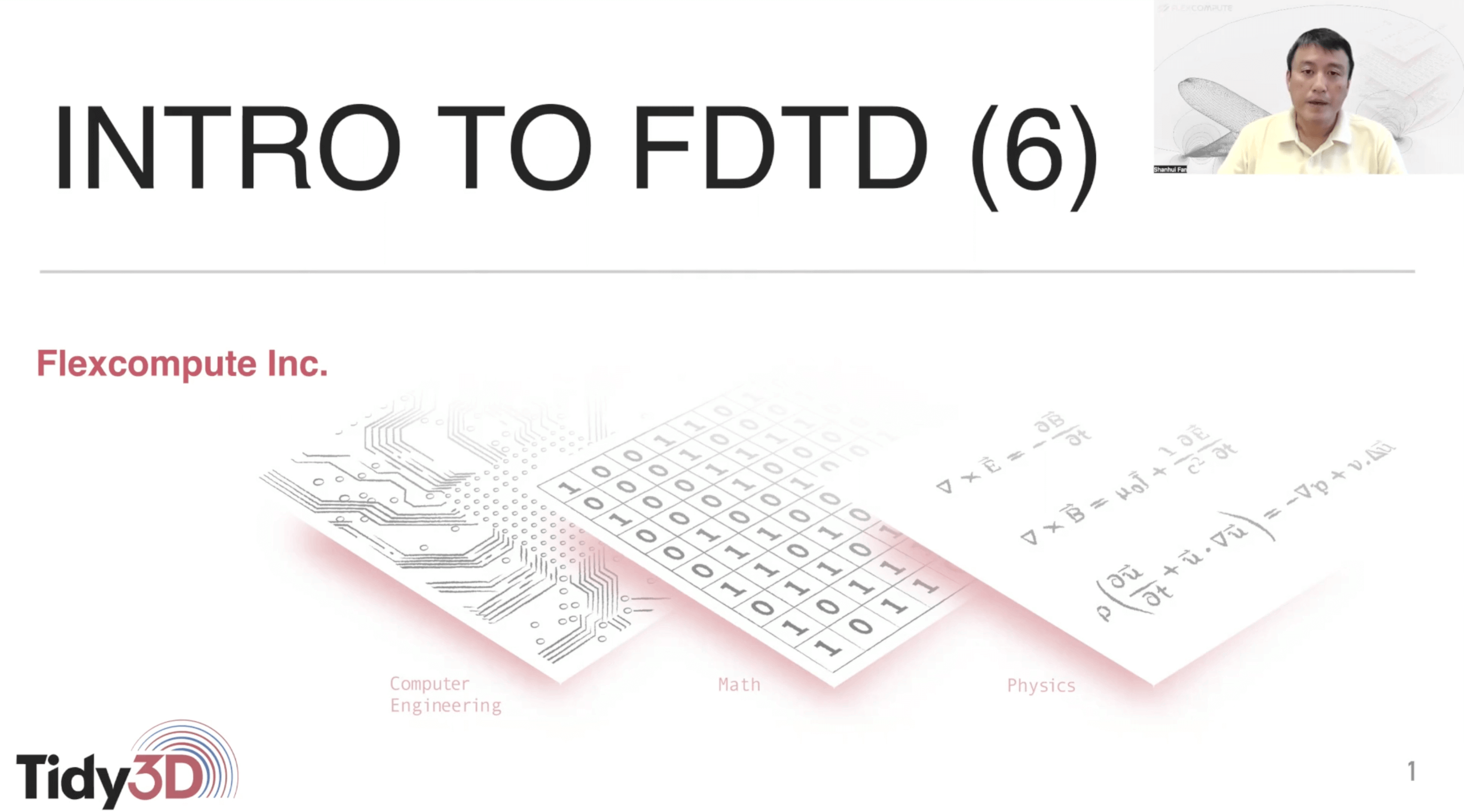## Lecture 6: Introduction to perfectly matched layer (PML)

Perfectly matched layer (PML) is commonly used to truncate unbounded computational region, since an ideal PML can completely absorb the incoming waves from all angles of the incidence without any reflection. In this lecture, we explain the basic idea behind PML, and show how to characterize the performance of PML.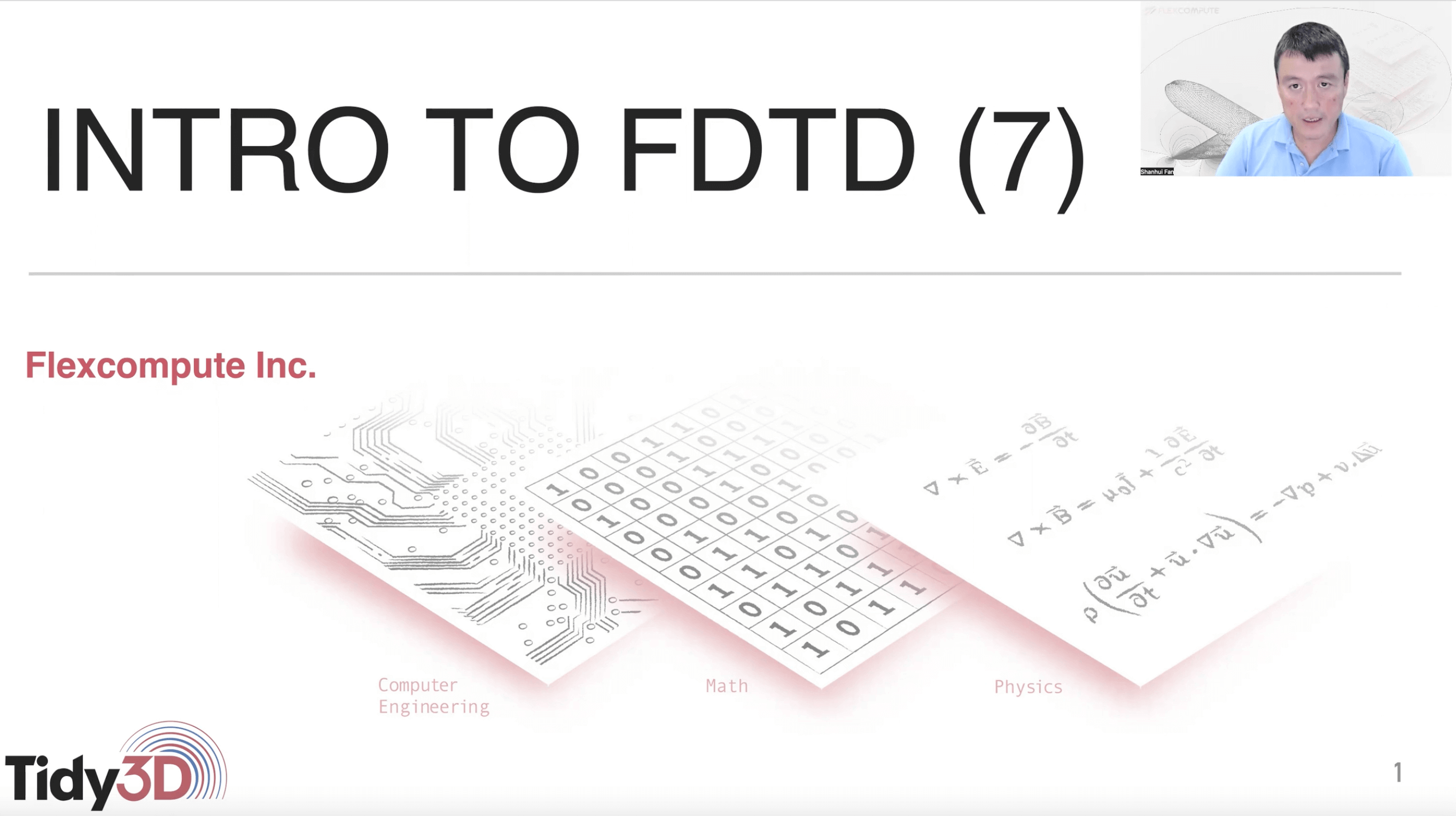## Lecture 7: Time step size and CFL condition in FDTD

The choice of time step size can have a strong impact on the behavior of FDTD algorithms. In this lecture, we provide a simple and intuitive argument on deriving an important condition on choosing time step size, known as the Courant–Friedrichs–Lewy (CFL) condition.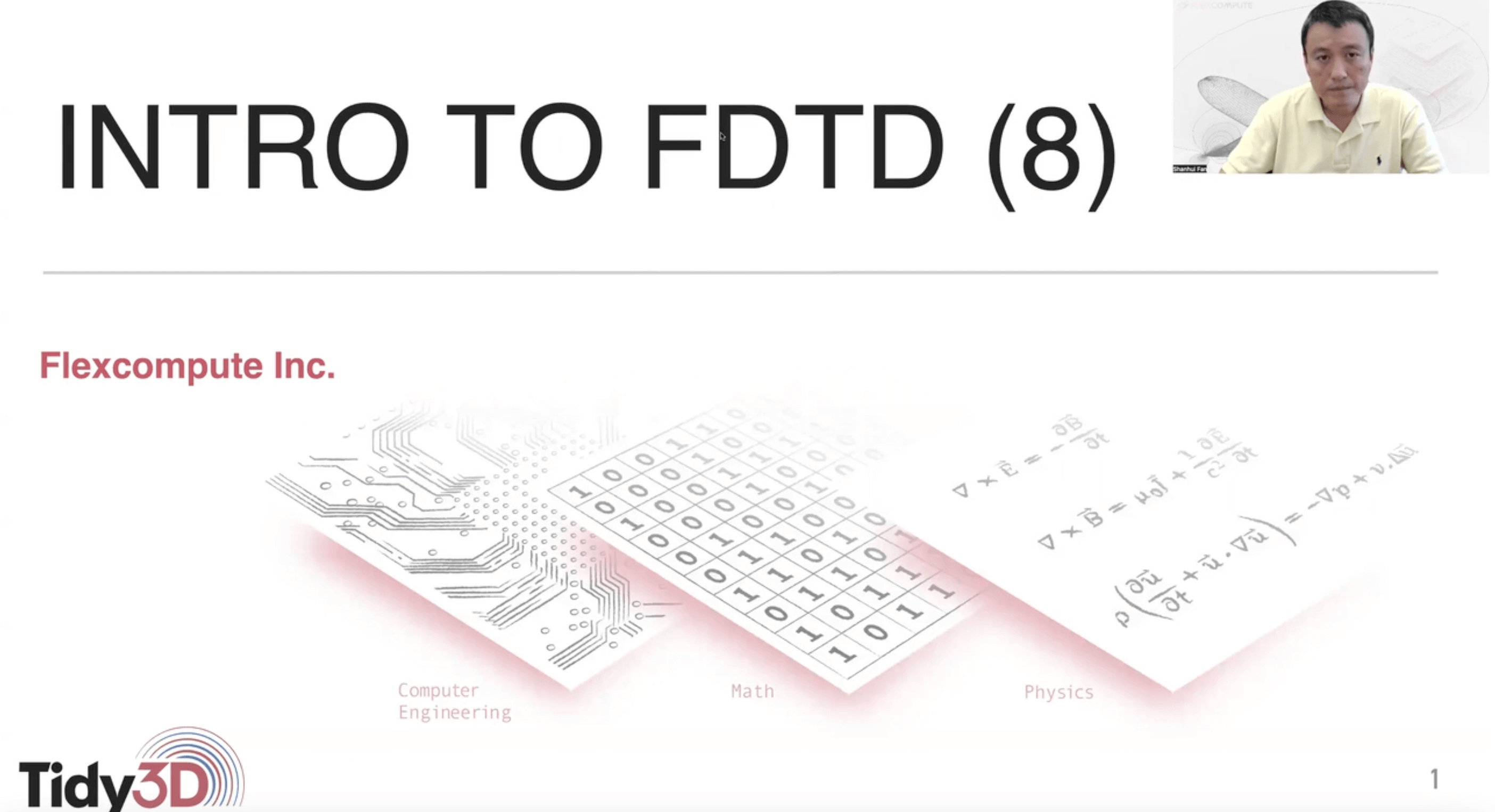## Lecture 8: Numerical dispersion in FDTD

A key source of error in FDTD simulations lies in the spatial and temporal discretization.The behavior of the wave propagating in such a discrete numerical lattice can deviatefrom that of the physical wave. This phenomenon is known as numerical dispersion. Inthis lecture, we derive and visualize the effect of numerical dispersion, as well asprovide a rule of thumb to suppress the error.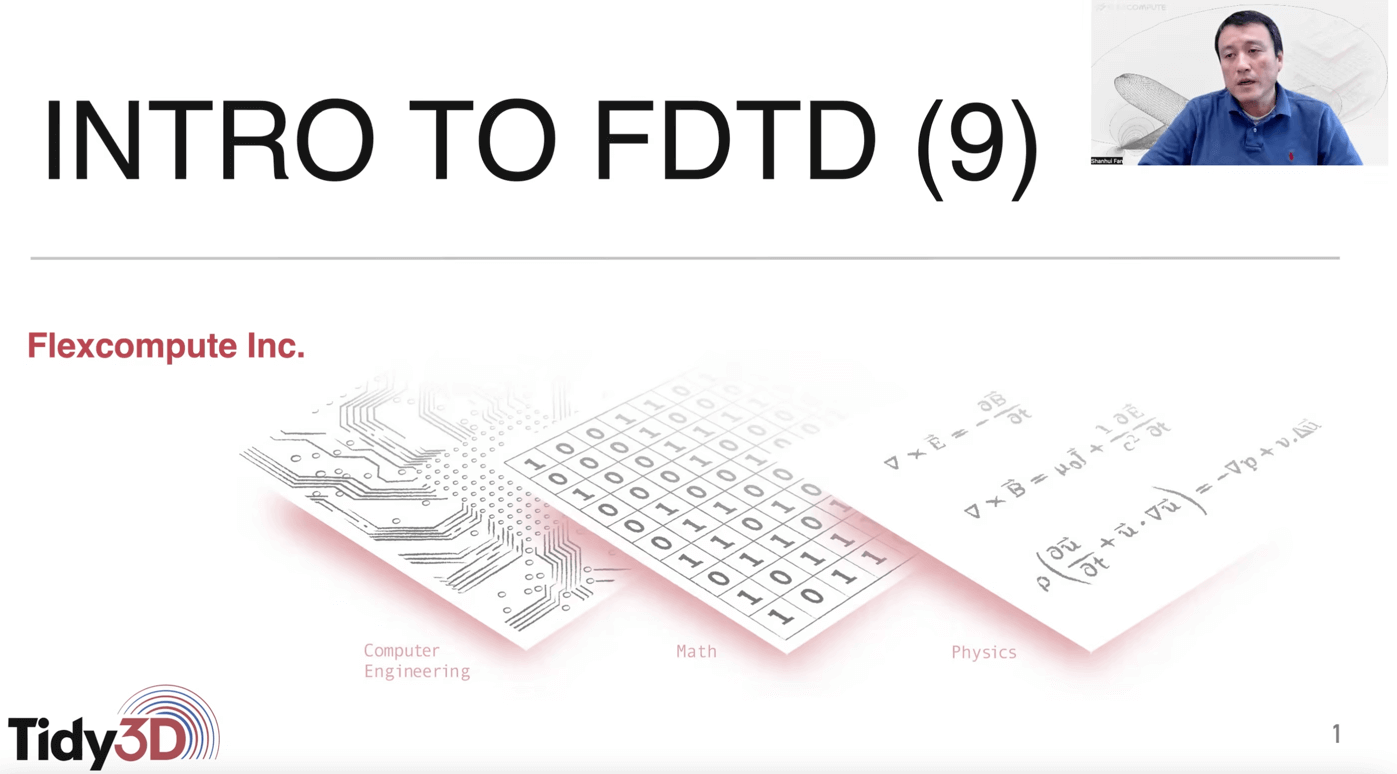## Lecture 9: Dielectric constant assignment on Yee grids

In this lecture, we discuss another important source of error that arises from the imperfect description of the dielectric constant distribution of a photonic device on discrete Yee grids. We highlight some subtleties in the dielectric constant assignment on the grids that can lead to significant error. This motivates us to consider more advanced FDTD techniques such as subpixel averaging and nonuniform grid sizes.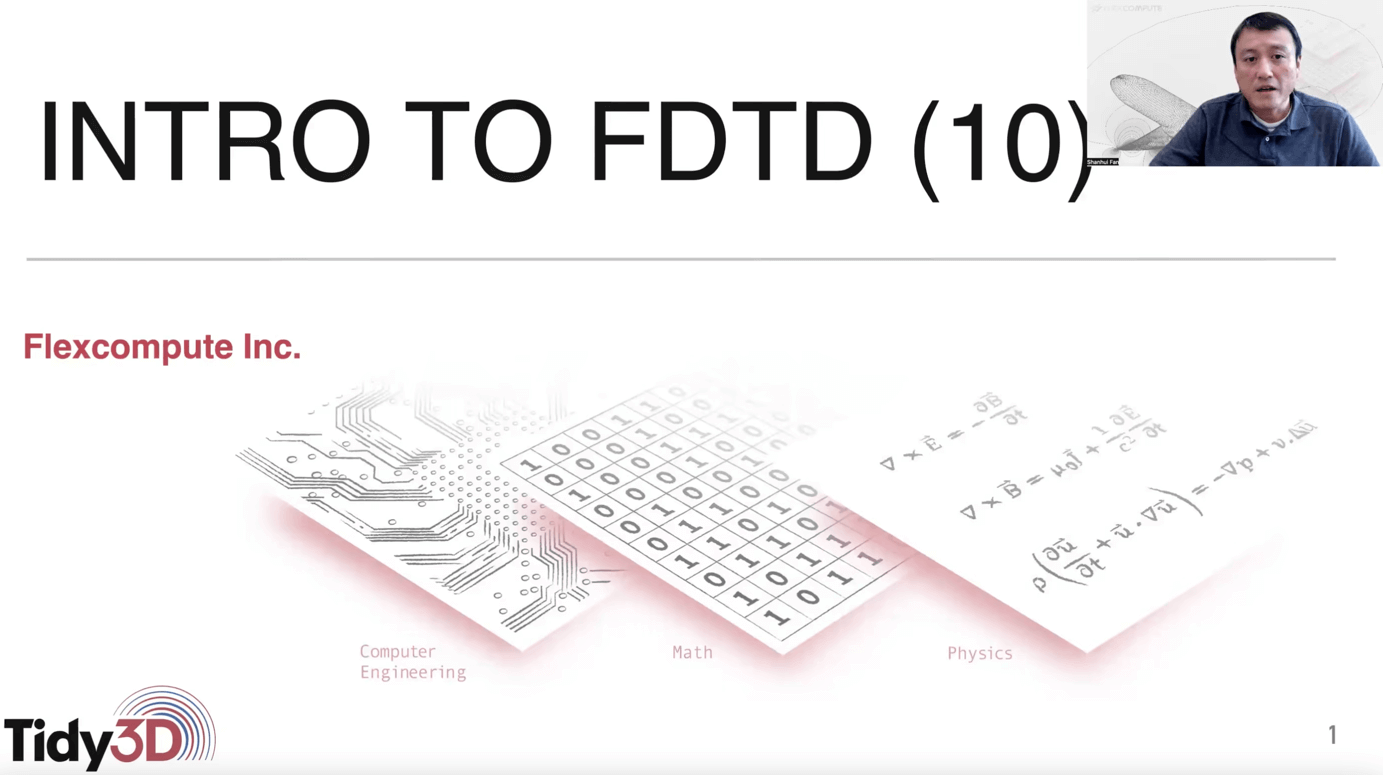## Lecture 10: Introduction to subpixel averaging

In this lecture, we discuss a powerful method known as subpixel averaging that enables the FDTD algorithm to capture geometric features below the discretization level. We discuss the basic ideas as well as some subtleties associated with subpixel averaging.